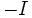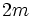# Symplectic group

## Definition

### In terms of a specific matrix description

For a positive integer$m$ and a field$k$, the symplectic group$Sp(2m,k)$ is defined as the group, under matrix multiplication, with the following underlying set:$\{ A \in GL(2m,k) \mid A\begin{pmatrix}0 & I \\ -I & 0 \\\end{pmatrix}A^T = \begin{pmatrix}0 & I \\ -I & 0 \\\end{pmatrix} \}$.

where$I$ and$-I$ are respectively the$n \times n$ identity and negative identity matrices.

The symplectic group$Sp(2m,k)$ is sometimes termed the symplectic group of degree$m$ and sometimes the symplectic group of degree$2m$.

### A more general description boiling down to the same thing

Suppose$k$ is a field and$b$ is a nondegenerate alternating bilinear form on a finite-dimensional vector space$V$ over$k$. The symplectic group for$b$ is defined as the group of all the linear transformations of$V$ that preserve$b$, i.e., maps$A$ satisfying:$\! b(v,w) = b(Av,Aw) \ \forall \ v,w \in V$.

Although this definition makes sense for any alternating bilinear form, we typically assume that the alternating bilinear form is nondegenerate.

It turns out that, up to change of basis, all nondegenerate alternating bilinear forms are equivalent, and the existence of such forms forces the space to be even-dimensional. Thus, the symplectic groups for any two nondegenerate alternating bilinear forms on vector spaces of the same dimension are isomorphic, and we can use the concrete definition provided above.

## Facts$Sp(2m,k)$ is a subgroup of the special linear group$SL(2m,k)$, and for$m = 1$, it is precisely equal to the special linear group of degree two, i.e.,$SL(2,k)$.